# RD Sharma Solutions Class 12 Probability Exercise 31.5

RD Sharma Solutions for Class 12 Maths Chapter 31 Probability Exercise 31.5 are available here for students to start preparations for their board exams. Students can utilise these solutions to learn the correct methodology for solving problems. This resource also helps clear any doubts pertaining to any question instantly. Further, students are encouraged to practise the RD Sharma Class 12 Solutions on a regular basis as it would yield good results in the exams. Download the PDF of the solutions to these exercise problems for free, from the links given below.

## Download PDF of RD Sharma Solutions for Class 12 Maths Chapter 31 Exercise 5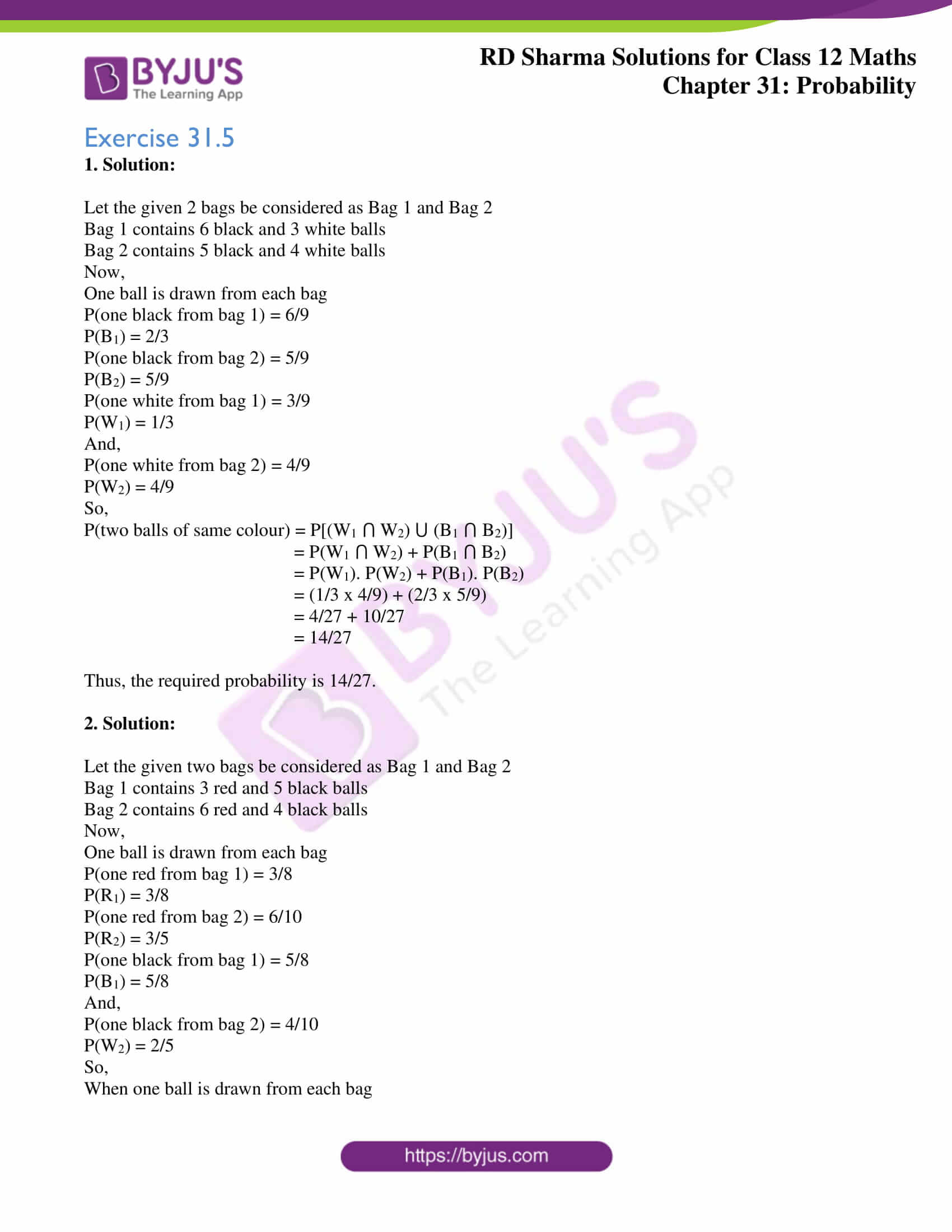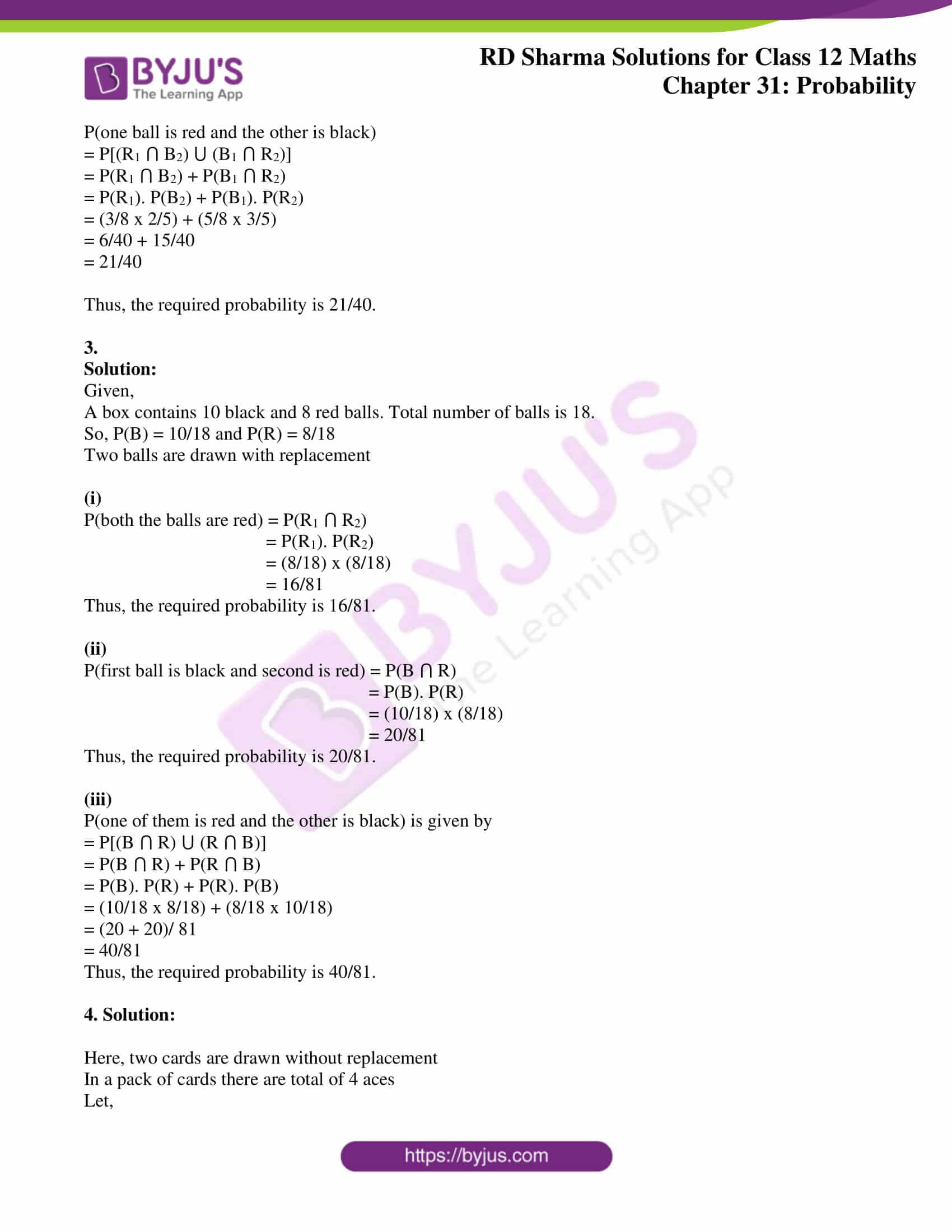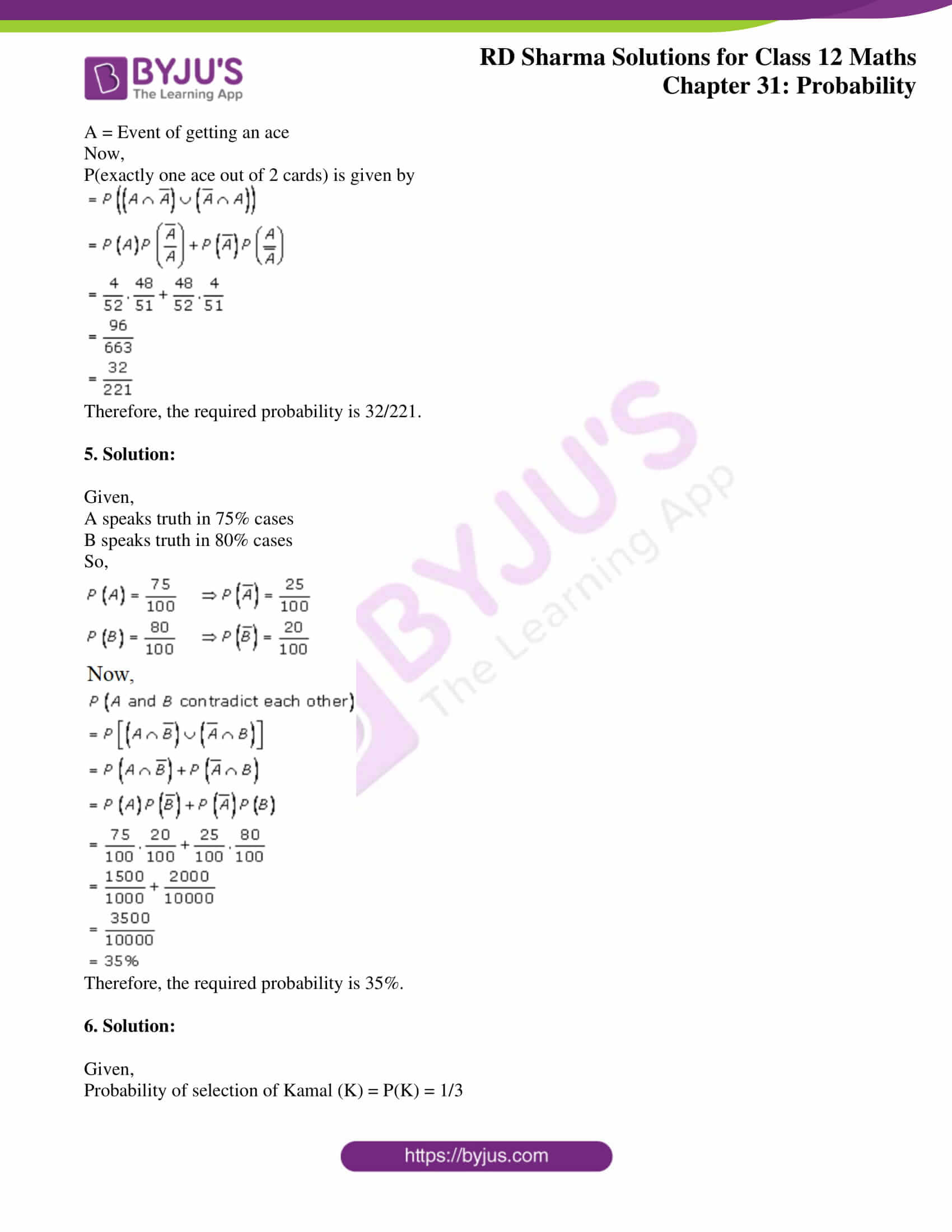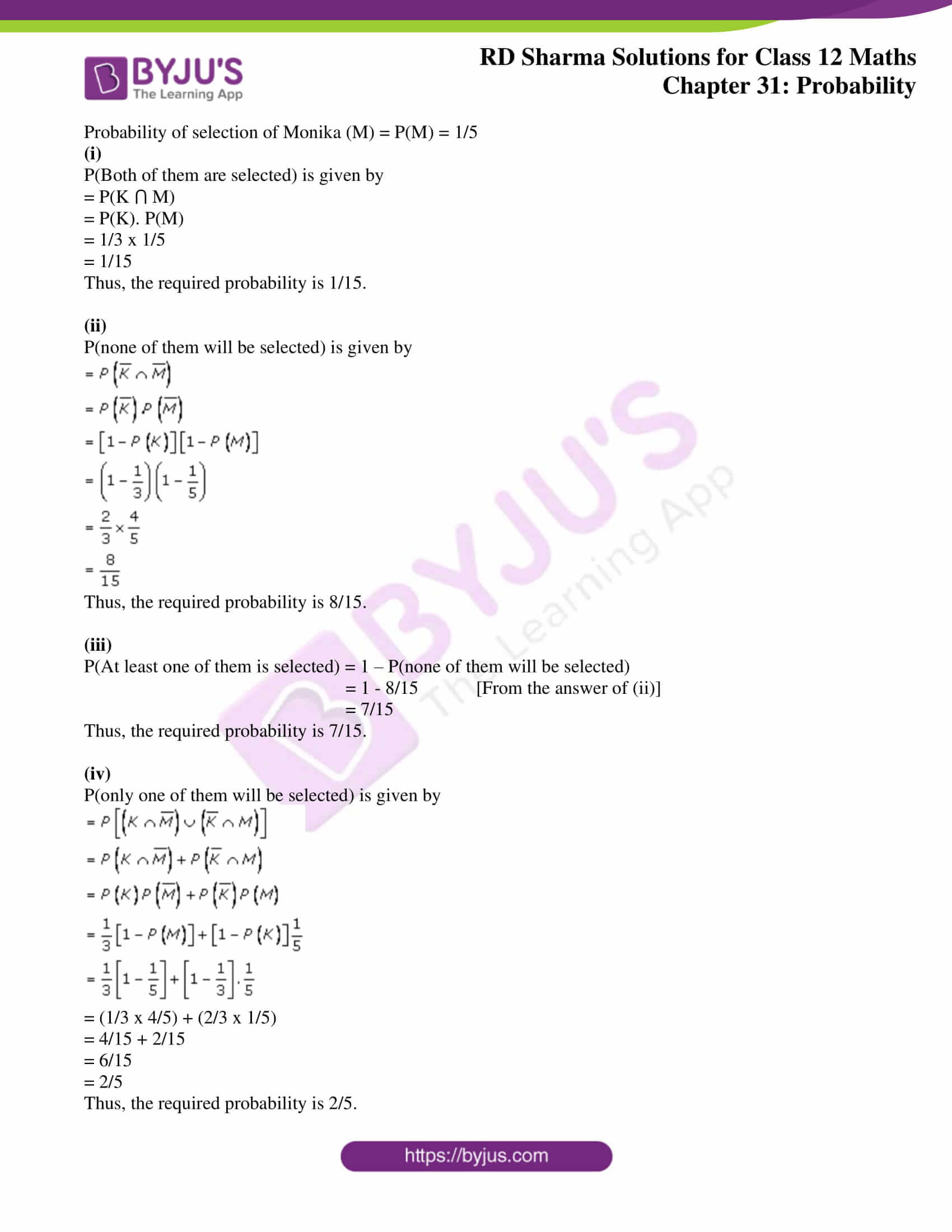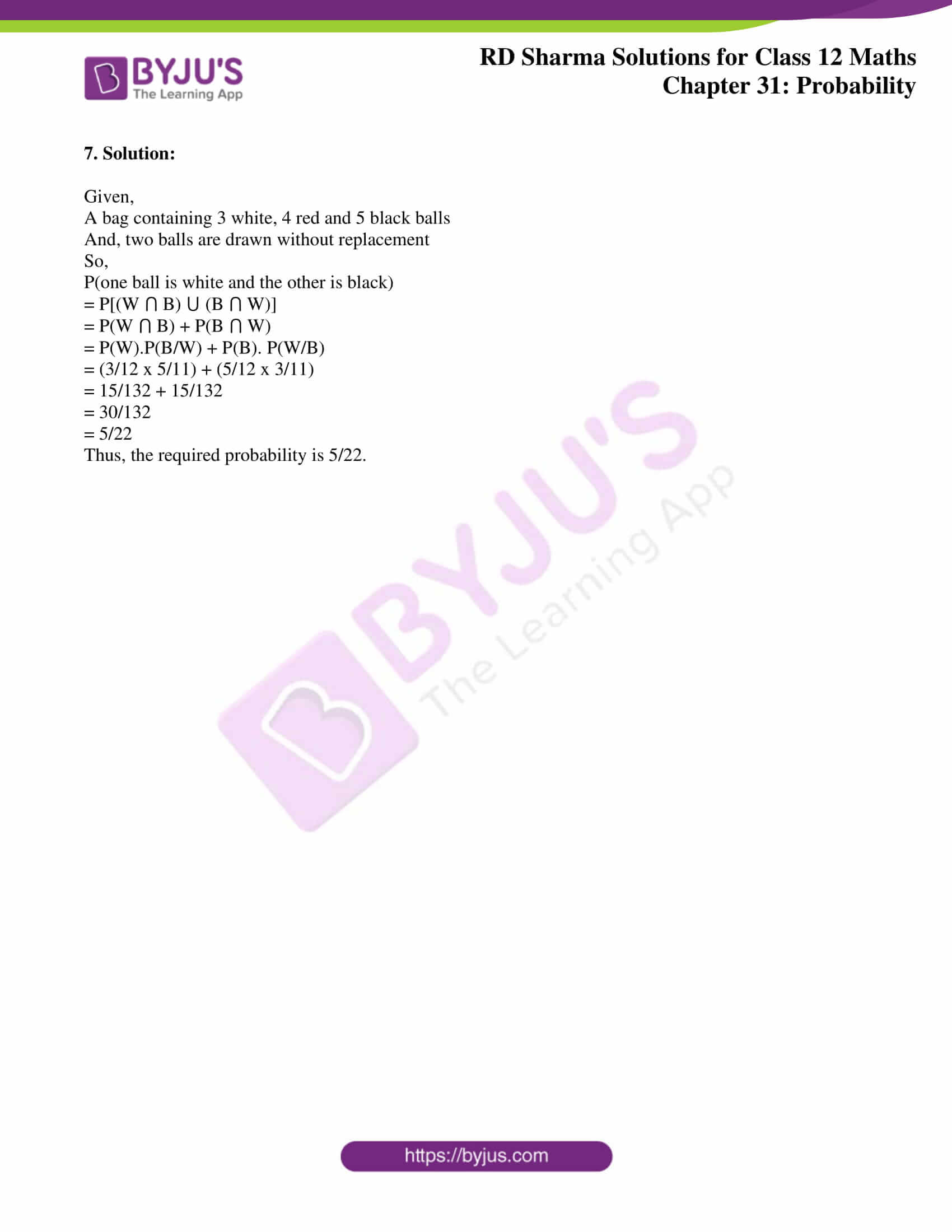### Access RD Sharma Solutions for Class 12 Maths Chapter 31 Exercise 5

Exercise 31.5

1. Solution:

Let the given 2 bags be considered as Bag 1 and Bag 2

Bag 1 contains 6 black and 3 white balls

Bag 2 contains 5 black and 4 white balls

Now,

One ball is drawn from each bag

P(one black from bag 1) = 6/9

P(B1) = 2/3

P(one black from bag 2) = 5/9

P(B2) = 5/9

P(one white from bag 1) = 3/9

P(W1) = 1/3

And,

P(one white from bag 2) = 4/9

P(W2) = 4/9

So,

P(two balls of same colour) = P[(W1 ⋂ W2) ⋃ (B1 ⋂ B2)]

= P(W1 ⋂ W2) + P(B1 ⋂ B2)

= P(W1). P(W2) + P(B1). P(B2)

= (1/3 x 4/9) + (2/3 x 5/9)

= 4/27 + 10/27

= 14/27

Thus, the required probability is 14/27.

2. Solution:

Let the given two bags be considered as Bag 1 and Bag 2

Bag 1 contains 3 red and 5 black balls

Bag 2 contains 6 red and 4 black balls

Now,

One ball is drawn from each bag

P(one red from bag 1) = 3/8

P(R1) = 3/8

P(one red from bag 2) = 6/10

P(R2) = 3/5

P(one black from bag 1) = 5/8

P(B1) = 5/8

And,

P(one black from bag 2) = 4/10

P(W2) = 2/5

So,

When one ball is drawn from each bag

P(one ball is red and the other is black)

= P[(R1 ⋂ B2) ⋃ (B1 ⋂ R2)]

= P(R1 ⋂ B2) + P(B1 ⋂ R2)

= P(R1). P(B2) + P(B1). P(R2)

= (3/8 x 2/5) + (5/8 x 3/5)

= 6/40 + 15/40

= 21/40

Thus, the required probability is 21/40.

3.

Solution:

Given,

A box contains 10 black and 8 red balls. Total number of balls is 18.

So, P(B) = 10/18 and P(R) = 8/18

Two balls are drawn with replacement

(i)

P(both the balls are red) = P(R1 ⋂ R2)

= P(R1). P(R2)

= (8/18) x (8/18)

= 16/81

Thus, the required probability is 16/81.

(ii)

P(first ball is black and second is red) = P(B ⋂ R)

= P(B). P(R)

= (10/18) x (8/18)

= 20/81

Thus, the required probability is 20/81.

(iii)

P(one of them is red and the other is black) is given by

= P[(B ⋂ R) ⋃ (R ⋂ B)]

= P(B ⋂ R) + P(R ⋂ B)

= P(B). P(R) + P(R). P(B)

= (10/18 x 8/18) + (8/18 x 10/18)

= (20 + 20)/ 81

= 40/81

Thus, the required probability is 40/81.

4. Solution:

Here, two cards are drawn without replacement

In a pack of cards there are total of 4 aces

Let,

A = Event of getting an ace

Now,

P(exactly one ace out of 2 cards) is given by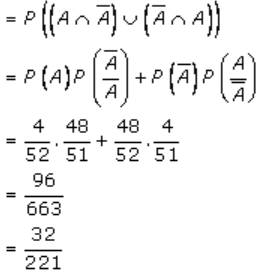Therefore, the required probability is 32/221.

5. Solution:

Given,

A speaks truth in 75% cases

B speaks truth in 80% cases

So,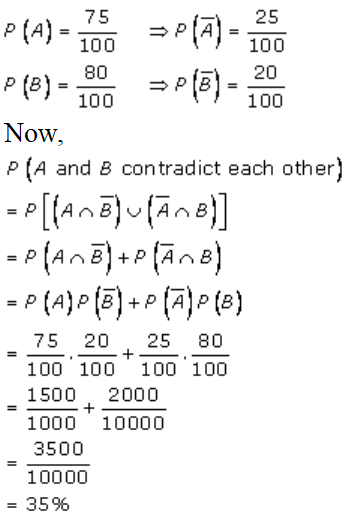Therefore, the required probability is 35%.

6. Solution:

Given,

Probability of selection of Kamal (K) = P(K) = 1/3

Probability of selection of Monika (M) = P(M) = 1/5

(i)

P(Both of them are selected) is given by

= P(K ⋂ M)

= P(K). P(M)

= 1/3 x 1/5

= 1/15

Thus, the required probability is 1/15.

(ii)

P(none of them will be selected) is given by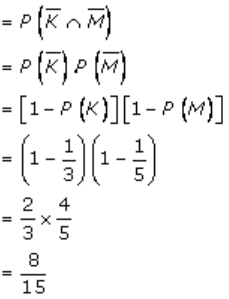Thus, the required probability is 8/15.

(iii)

P(At least one of them is selected) = 1 – P(none of them will be selected)

= 1 – 8/15 [From the answer of (ii)]

= 7/15

Thus, the required probability is 7/15.

(iv)

P(only one of them will be selected) is given by= (1/3 x 4/5) + (2/3 x 1/5)

= 4/15 + 2/15

= 6/15

= 2/5

Thus, the required probability is 2/5.

7. Solution:

Given,

A bag containing 3 white, 4 red and 5 black balls

And, two balls are drawn without replacement

So,

P(one ball is white and the other is black)

= P[(W ⋂ B) ⋃ (B ⋂ W)]

= P(W ⋂ B) + P(B ⋂ W)

= P(W).P(B/W) + P(B). P(W/B)

= (3/12 x 5/11) + (5/12 x 3/11)

= 15/132 + 15/132

= 30/132

= 5/22

Thus, the required probability is 5/22.